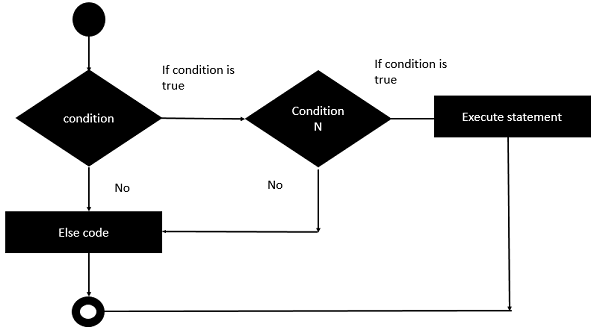# Rexx - Decision Making

Decision making structures require that the programmer specify one or more conditions to be evaluated or tested by the program.

The following diagram shows the general form of a typical decision-making structure found in most of the programming languages.There is a statement or statements to be executed if the condition is determined to be true, and optionally, other statements to be executed if the condition is determined to be false.

Let’s look at the various decision-making statements available in Rexx.

Sr.No. Statement & Description
1 If statement

The first decision-making statement is the if statement. An if statement consists of a Boolean expression followed by one or more statements.

2 If-else statement

The next decision-making statement is the if-else statement. An if statement can be followed by an optional else statement, which executes when the Boolean expression is false.

## Nested If Statements

Sometimes there is a requirement to have multiple if statements embedded inside each other, as is possible in other programming languages. In Rexx also this is possible.

### Syntax

```if (condition1) then
do
#statement1
end
else
if (condition2) then
do
#statement2
end
```

### Flow Diagram

The flow diagram of nested if statements is as follows −Let’s take an example of nested if statement −

### Example

```/* Main program */
i = 50
if (i < 10) then
do
say "i is less than 10"
end
else
if (i < 7) then
do
say "i is less than 7"
end
else
do
say "i is greater than 10"
end
```

The output of the above program will be −

```i is greater than 10
```

## Select Statements

Rexx offers the select statement which can be used to execute expressions based on the output of the select statement.

### Syntax

The general form of this statement is −

```select
when (condition#1) then
statement#1

when (condition#2) then
statement#2
otherwise

defaultstatement
end
```

The general working of this statement is as follows −

• The select statement has a range of when statements to evaluate different conditions.

• Each when clause has a different condition which needs to be evaluated and the subsequent statement is executed.

• The otherwise statement is used to run any default statement if the previous when conditions do not evaluate to true.

### Flow Diagram

The flow diagram of the select statement is as followsThe following program is an example of the case statement in Rexx.

### Example

```/* Main program */
i = 50
select
when(i <= 5) then
say "i is less than 5"

when(i <= 10) then
say "i is less than 10"

otherwise
say "i is greater than 10"
end
```

The output of the above program would be −

```i is greater than 10
```